ISEE Lower Level Quantitative : How to find the probability of an outcome

Example Questions

← Previous 1 3 4 5

Example Question #1 : How To Find The Probability Of An Outcome

Eric has a 2 red shirts, 3 orange shirts, 4 purple shirts, and 1 pink shirt.  If Eric randomly chooses a shirt, what are the chances that he selects a pink shirt?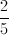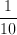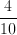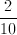Explanation:

First, add up all of the shirts.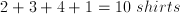We then see that Eric has 1 pink shirt and 10 shirts from which to choose.  Build a fraction with the number of pink shirts on top, and the total number of shirts on the bottom,.

Example Question #2 : Data Analysis And Probability

Casey has a bag containing red, yellow, blue, and green beads.  There are equal numbers of red and blue beads, which comprise half the bag when combined.  If there are 24 beads in the bag, what is the probability that Casey will select a blue bead?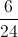Explanation:

There are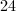beads in Casey's bag.  We know that the red and blue beads make up half of the bag, so we divideby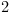to find the total number of red and blue beads.Since there is an equal number of red and blue beads, we divide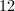by.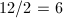To find probability, we create a fraction with our desired outcome written as the numerator and the total possible outcomes written as the denominator: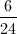Since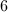andare divisible by, we must reduce the fraction.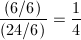Example Question #2 : How To Find The Probability Of An Outcome

There are 2 blue blocks, 3 red blocks, and some yellow blocks on the ground. If the probability of picking a red block is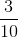, how many yellow blocks are there?Explanation:

The probability given in the problem tells us how many total blocks there are, which is 10. (Probability is part over whole.) Since we know that there are 10 total, we can subtract the quantities of the red and blue blocks: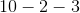Therefore there are 5 yellow blocks.

Example Question #1 : Outcomes

A couple wants to have two children. If they have a 50% chance of having a boy and 50% chance of having a girl for each birth, what is the chance that they will eventually have one boy and one girl?Explanation:

If a couple has a 50% chance of having a boy and 50% chance of having a girl for each birth, below are the four possible outcomes of their children:

First a girl, then a boy.

First a girl, then another girl.

First a boy, then another boy.

First a boy, then a girl.

In 2 of these 4 scenarios, there will be one boy and one girl. Therefore, the chance of having one boy and one girls is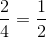.

Example Question #1 : Probability

Brett is taking a multiple choice test in which there are 3 answer choices. He blindly guesses on 2 of the problems. What is the chance that he guesses correctly on both?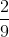Explanation:

If Brett blindly guesses on a multiple choice question with 3 answer choices, he has achance of getting it correct.

If he guesses on 2 multiple choice questions with 3 answer choices each, then the probability of him getting them both correct is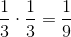.

Example Question #6 : Data Analysis And Probability

If Marie rolls a die with six sides, what is the probability that it will land on a prime number?Explanation:

If Marie rolls a die, the possible number that will appear are: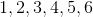Of these numbers, 2, 3, and 5 are prime. Three out of the six numbers are prime, so the probability of landing on a prime number is.

Example Question #1 : Data Analysis And Probability

Ten cards each have a number printed on them. Five have a 1 printed on them, three have a 2, and two have a 3. The cards are shuffled and a card is dealt. What is the probability that the card will not be a 3?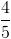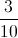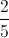Explanation:

There are ten cards total, and eight cards that are not threes. This makes the probability of drawing a three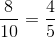Example Question #1 : How To Find The Probability Of An Outcome

If there are 2 blue marbles and 18 red marbles in a jar, what is the probability that Jeff will pick out a red marble?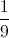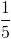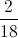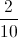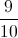Explanation:

First, add the total number of marbles, which is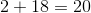. There are 18 red marbles, so you set up a fraction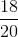.

If you simplify by dividing the numerator and denominator by 2, you get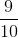.

Example Question #3 : Probability

A magician holds in his bag 3 green balls, 4 red balls and 7 blue balls. What is the probability of drawing a red ball from the bag?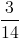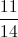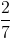Explanation:

Probability = total number of possible outcomes/sample space.

=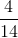=Example Question #4 : Probability

Find the probability of an outcome. Express the outcome as fraction, reduced to its lowest terms.

Mr. Thomas went to a car lot to buy a new car. Of the 68 cars on the lot, 22 were black, 16 were silver, 8 were white, 12 were blue, and 10 were red.  What is the probability that Mr. Thomas will buy a blue car?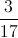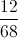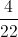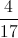Explanation:

To find the probability of an outcome, set up a fraction: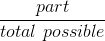Since there are 12 blue cars (part) out of 68 cars in all (total possible) the fraction looks like this: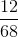If you reduce the fraction by 4 (because the numerator and the denominator are both divisible by 4 which makes 4 the GCF (greatest common factor), it becomes: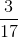← Previous 1 3 4 5